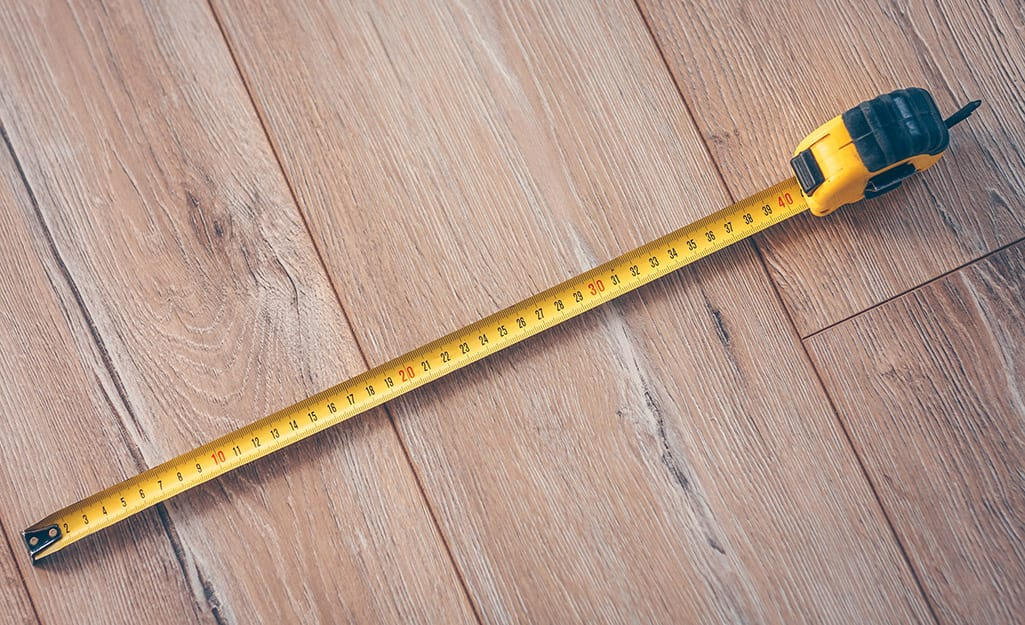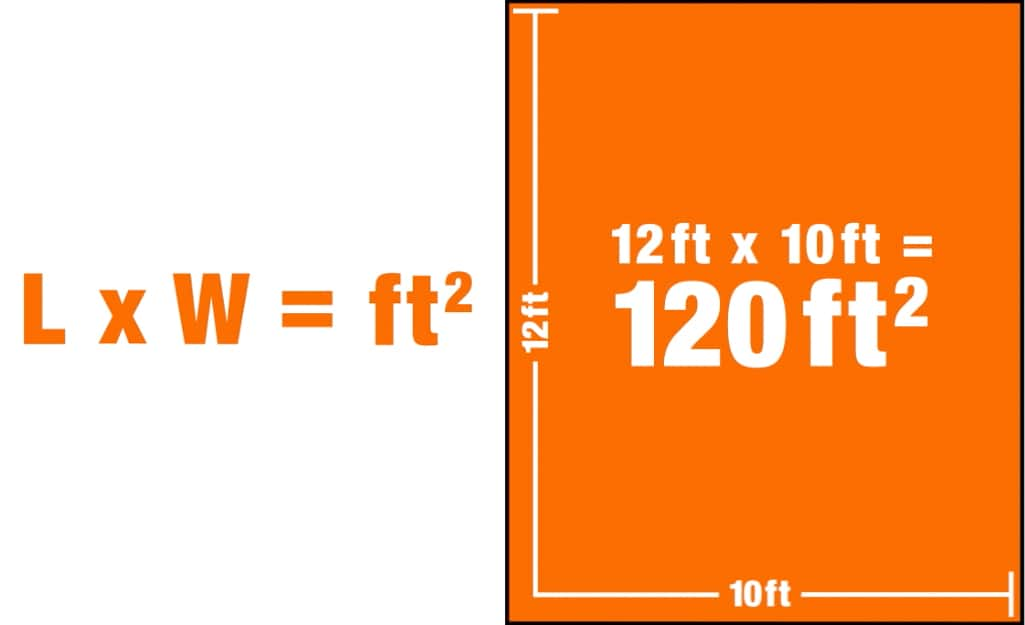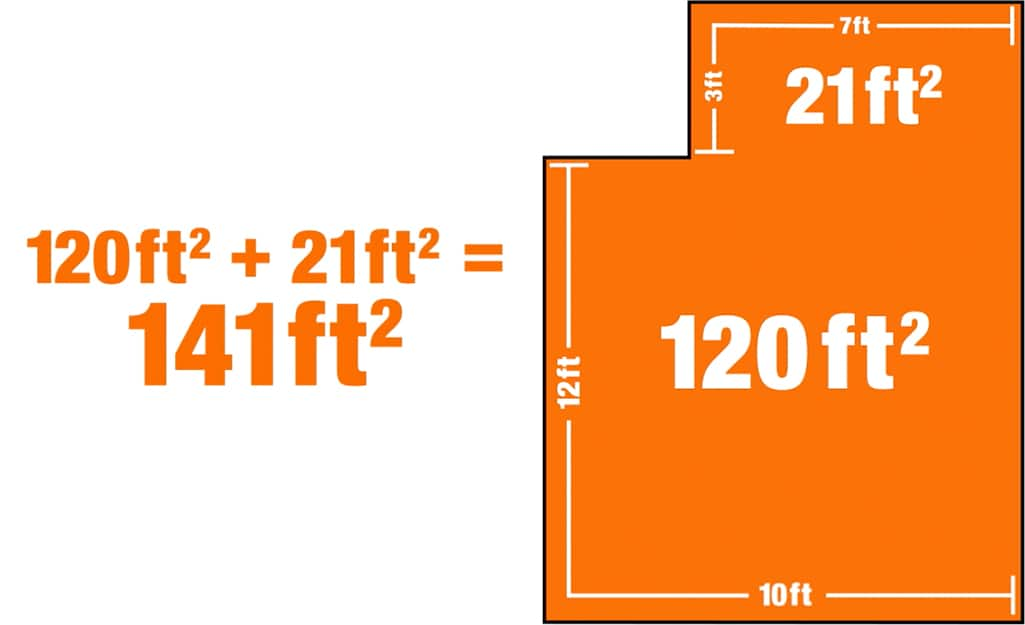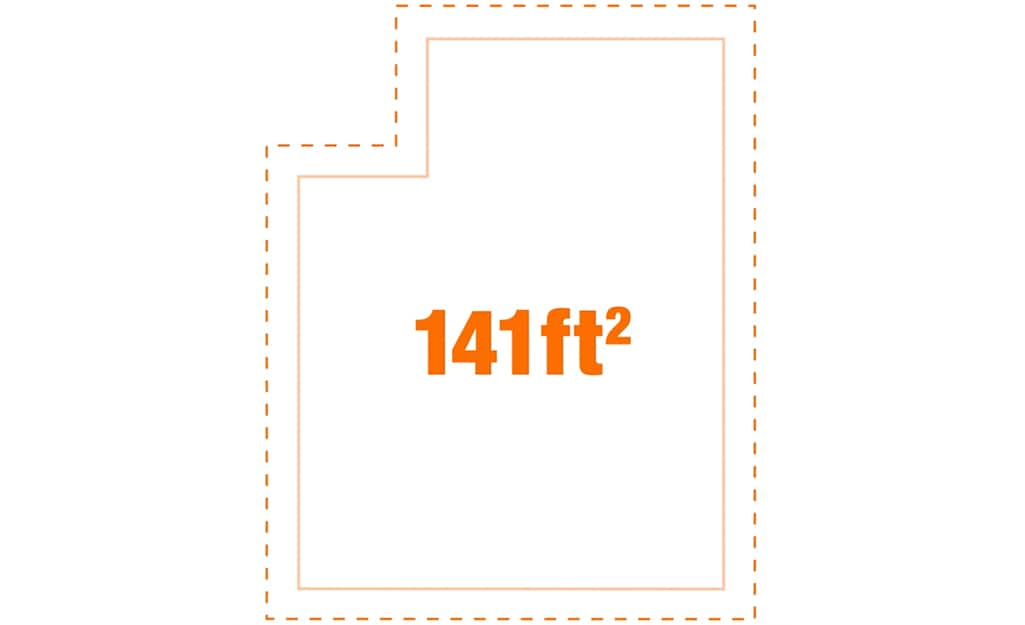#1 Home Improvement Retailer

# How to Calculate Square Footage

## Tools## Materials

Measure the AreaTo calaculate room square footage, first measure the dimensions of your space. The two dimensions to measure are the length and width of the area you need to calculate.

• To find length, locate the longest side of the area to be measured.
• Fix a tape measure or other measuring tool to one end of the length and extend it to the other end.
• Record the measurement.
• To find the width, locate the shortest side of the area to be measured. Repeat the process and record that measurement.
Apply the Square Footage FormulaThe next step in how to calculate square footage is to plug your measurements into the square footage formula: L x W = A (in square feet).

To find square feet, multiply the length measurement in feet by the width measurement in feet. This yields a product called the area, which is expressed in square feet (or square inches if you are calculating a much smaller space, such as a dollhouse).

For example, if you are buying carpet for a room that is 12-feet long and 10-feet wide, multiply the two dimensions:

• 12 ft. x 10 ft. = 120 sq. ft.
Account for Odd DimensionsSometimes you will have to account for odd room dimensions or additional areas that don't neatly connect with your main room. In this case, to calculate the square footage accurately, you may need to divide the space into separate areas.

• Divide the space into smaller sections.
• Measure each section separately and calculate the square footage of each.
• Use a calculator to add the numbers together to get the total square footage.

For example, assume you are trying to buy flooring for a rectangular room with a nook. The shape is of a large rectangle and a smaller rectangle.

• Find the length and width of each section (labeled A and B here), then calculate each square footage:
A: 3 ft. x 7 ft. = 21 sq. ft.
B: 12 ft. x 10 ft. = 120 sq. ft.

• Then, add the three values together to get the total square footage you’ll need:
120 sq. ft. + 21 sq. ft. = 141 sq. ft.

• Therefore, you’ll need 141 sq. ft. of flooring materials.